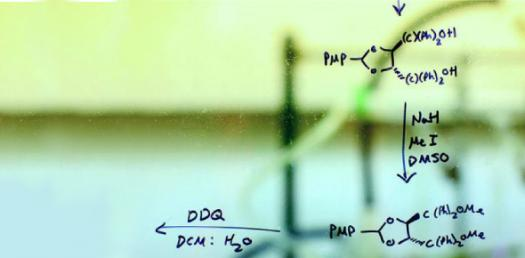# Chemistry Trivia Challenge! Hardest Questions Quiz

49 Questions | Total Attempts: 35SettingsRelated Topics
• 1.
What is this lab safety symbol warning a person of?
• A.

Biohazard: Plant that is toxic to humans

• B.

Biohazard: Bacteria or Virus that could be transmitted

• C.

• D.

Electrical Safety: Electrical shock could harm or kill you

• 2.
Which of the following is NOT matched correctly with what they measure?
• A.

Triple Beam Balance - Mass (grams)

• B.

• C.

Test Tube - Volume (liters)

• D.

Ruler - Length (Centimeters)

• 3.
Which of the following would INCREASE the validity in a study if Vitamin C makes wounds heal faster?
• A.

Using few people per study group

• B.

Giving each group of people different levels of Vitamin C

• C.

Make groups with all different severities of wounds

• D.

Make groups with people all around the same age

• 4.
Based on the graph provided, which pH is the best (optimal) pH for tadpoles?
• A.

8

• B.

7.5

• C.

7

• D.

6.5

• E.

6

• F.

5.5

• 5.
Jay broke a test tube in the lab but was not injured in the process of breaking the test tube or sweeping it up. Where should he put the broken test tube?
• A.

Trashcan

• B.

Biohazard Container

• C.

Safety Shower

• D.

Broken Glass Container

• 6.
What is the independent variable in the following study: A study that tests if more time studying will improve test scores.
• A.

Test Scores

• B.

Number of participants per Group

• C.

One group that does not study at all

• D.

Amount of time studying

• 7.
Lead (Pb-208) is the heaviest stable isotope known. It has an atomic number of 82. How many neutrons does Pb-208 have?
• A.

82

• B.

126

• C.

164

• D.

208

• 8.
What are found in the space surrounding the nucleus of an atom?
• A.

Protons

• B.

Electrons

• C.

Neutrons

• D.

Ions

• 9.
If an atom gains an electron, what will be the overall charge of the atom?
• A.

Neutral

• B.

Positive

• C.

Negative

• D.

• 10.
What bonds are formed by water molecules attracting to other water molecules through a process called cohesion?
• A.

Polar

• B.

Covalent

• C.

Ionic

• D.

Hydrogen

• 11.
What is the monomer of nucleic acids?
• A.

Monosaccharides

• B.

Nucleotides

• C.

Fatty acids

• D.

Amino acids

• 12.
A map of eastern North America, showing the pH of rainfall in the various states, indicates that the pH of rain in New York State varies from 4.22 to 4.40. According to these figures, the most acidic rainfall in New York State has a pH of
• A.

4.22

• B.

4.30

• C.

4.35

• D.

4.40

• 13.
What is the function of enzymes?
• A.

Short term energy storage

• B.

Long term energy storage

• C.

Speeds up the rate of chemical reactions

• D.

Stores genetic information

• 14.
Which of the following structures would indicate that a cell is NOT prokaryotic?
• A.

Cell membrane

• B.

Mitochondria

• C.

DNA

• D.

Ribosome

• 15.
Which of the following would indicate that the cell you are observing is a plant cell rather than an animal cell?
• A.

Ribosomes

• B.

Cell Membrane

• C.

Nucleus

• D.

Cell Wall

• 16.
What is the letter 'N' pointing to?
• A.

Endoplasmic Reticulum

• B.

Golgi Apparatus

• C.

Mitochondria

• D.

Nucleus

• 17.
What is the function of the phospholipid bilayer?
• A.

Control what goes into and out of the cell

• B.

Provides energy for the cell

• C.

Helps the cell move around

• D.

Store excess materials in the cell

• 18.
If a cell has 5% dissolved solutes, and the solution that has 3% dissolved solutes, what kind of solution is the cell in?
• A.

Hypertonic

• B.

Hypotonic

• C.

Isotonic

• 19.
What is the function of ribosomes?
• A.

To store genetic information

• B.

To help the cell move

• C.

To make proteins

• D.

To move things in and out of the cell

• 20.
Which of the following is NOT a true statement about ATP
• A.

ATP consists of ribose, adenine, and three phosphate groups

• B.

ADP is produced when ATP releases energy

• C.

ATP provides energy for the mechanical functions of cells

• D.

Used ATP is discarded by the cell as waste

• 21.
Which of the following is an autotroph?
• A.

Rabbit

• B.

Grasshopper

• C.

Oak Tree

• D.

Fruit Fly

• 22.
Where do plants get the energy they need to carry out photosynthesis?
• A.

Air

• B.

Soil

• C.

Sunlight

• D.

Water

• 23.
Which of the following is showing ATP in this Photosynthesis reaction?
• A.

4

• B.

5

• C.

6

• D.

7

• 24.
What is the item labeled 'D' in the diagram of cellular respiration?
• A.

Glucose

• B.

Oxygen

• C.

Water

• D.

Carbon Dioxide

• 25.
Which of the following shows the steps of cellular respiration in the correct order?
• A.

Fermentation, Glycolysis, Electron Transport Chain

• B.

Electron Transport Chain, Krebs Cycle, Glycolysis

• C.

Glycolysis, Electron Transport Chain, Krebs Cycle

• D.

Glycolysis, Krebs Cycle, Electron Transport Chain